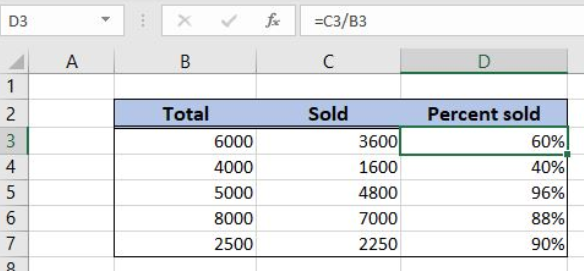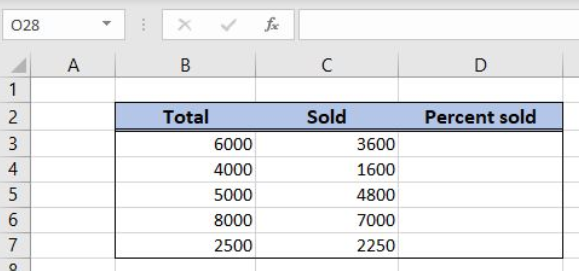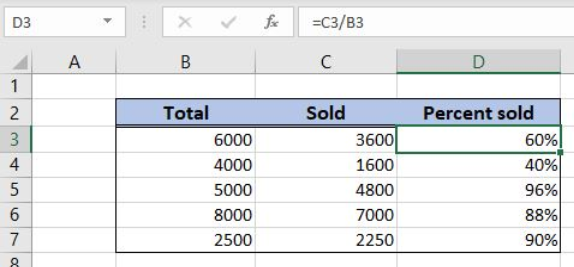Get instant live expert help with Excel or Google Sheets“My Excelchat expert helped me in less than 20 minutes, saving me what would have been 5 hours of work!”

#### Post your problem and you'll get expert help in seconds

Your message must be at least 40 characters
Our professional experts are available now. Your privacy is guaranteed.

# Percent sold

Excel allows a user to get a percent of sold products using the simple division. This step by step tutorial will assist all levels of Excel users in getting a percent sold from a total.Figure 1. The result of the formula

## Syntax of the Formula

The generic formula is:

`=total / sold`

The parameters of the formula are:

• total – a total amount
• sold – a sold amount from total

## Setting up Our Data for the Formula

Let’s look at the structure of the data we will use. In column B (“Total”), we have a total amount and in column C (“Sold”) we have a sold amount. In the column D (“Percent sold”), we want to get a percentage of the sold amount.Figure 2. Data that we will use in the example

## Get a Percentage of a Sold Amount

In our example, we want to get a percentage of a sold amount (3600). The result will be in the cell D3.

The formula looks like:

`=B3/C3`

The parameter total is the cell B3, while the parameter sold is C3.

To apply the formula, we need to follow these steps:

• Select cell D3 and click on it
• Insert the formula: `=B3/C3`
• Press enter
• Drag the formula down to the other cells in the column by clicking and dragging the little “+” icon at the bottom-right of the cell.Figure 3. Using the formula to get a percentage of a sold amount

Finally, the result in the cell D3 is 60%.

Most of the time, the problem you will need to solve will be more complex than a simple application of a formula or function. If you want to save hours of research and frustration, try our live Excelchat service! Our Excel Experts are available 24/7 to answer any Excel question you may have. We guarantee a connection within 30 seconds and a customized solution within 20 minutes.

### Did this post not answer your question? Get a solution from connecting with the expert.Another blog reader asked this question today on Excelchat:## Subscribe to Excelchat.coAnother blog reader asked this question today on Excelchat: